Past Year - 3 Mark Questions

Class 10
Chapter 12 Class 10 - Electricity

## Show how would you join three resistors, each of resistance 9 W so that the equivalent resistance of the combination is (i) 13.5 W, (ii) 6 W?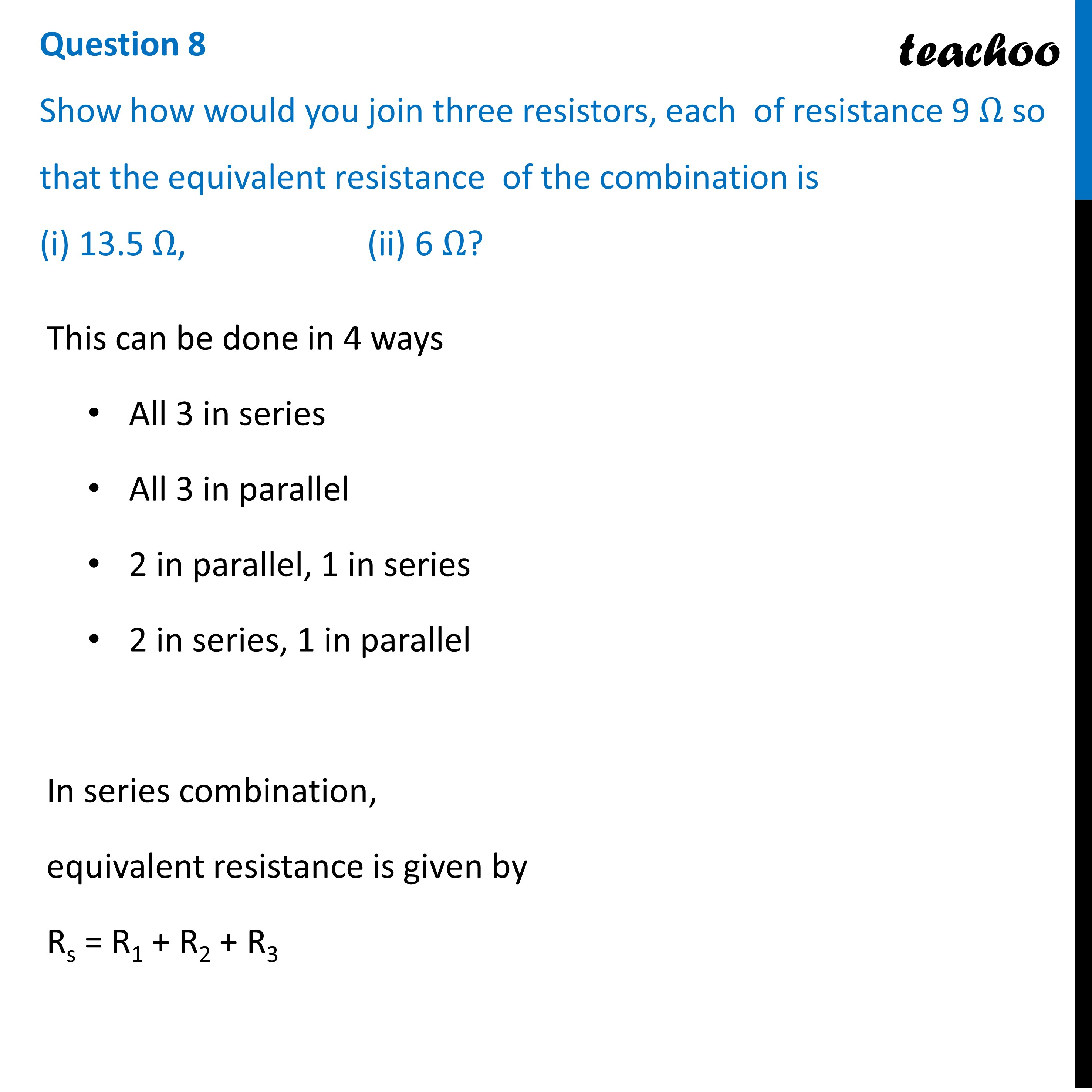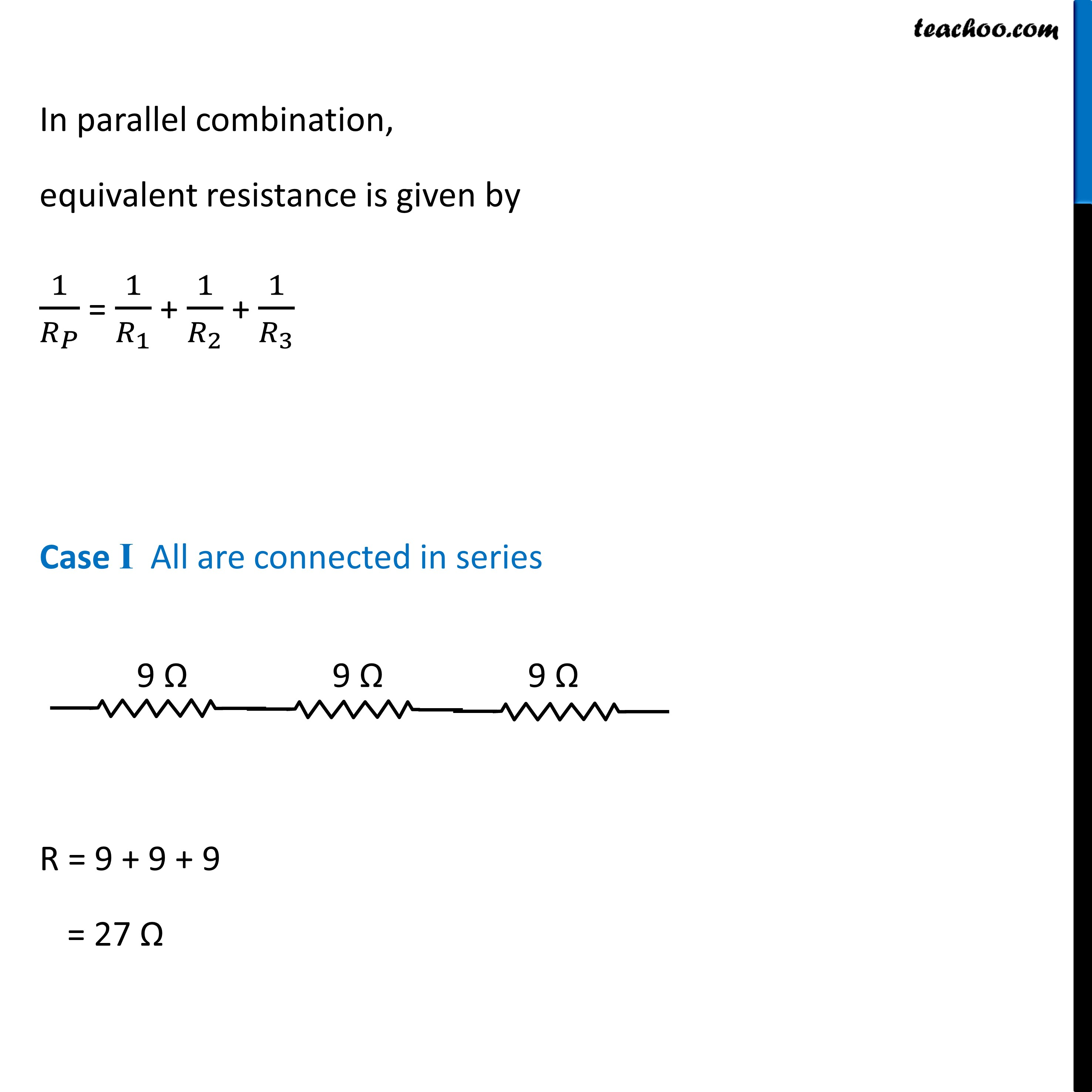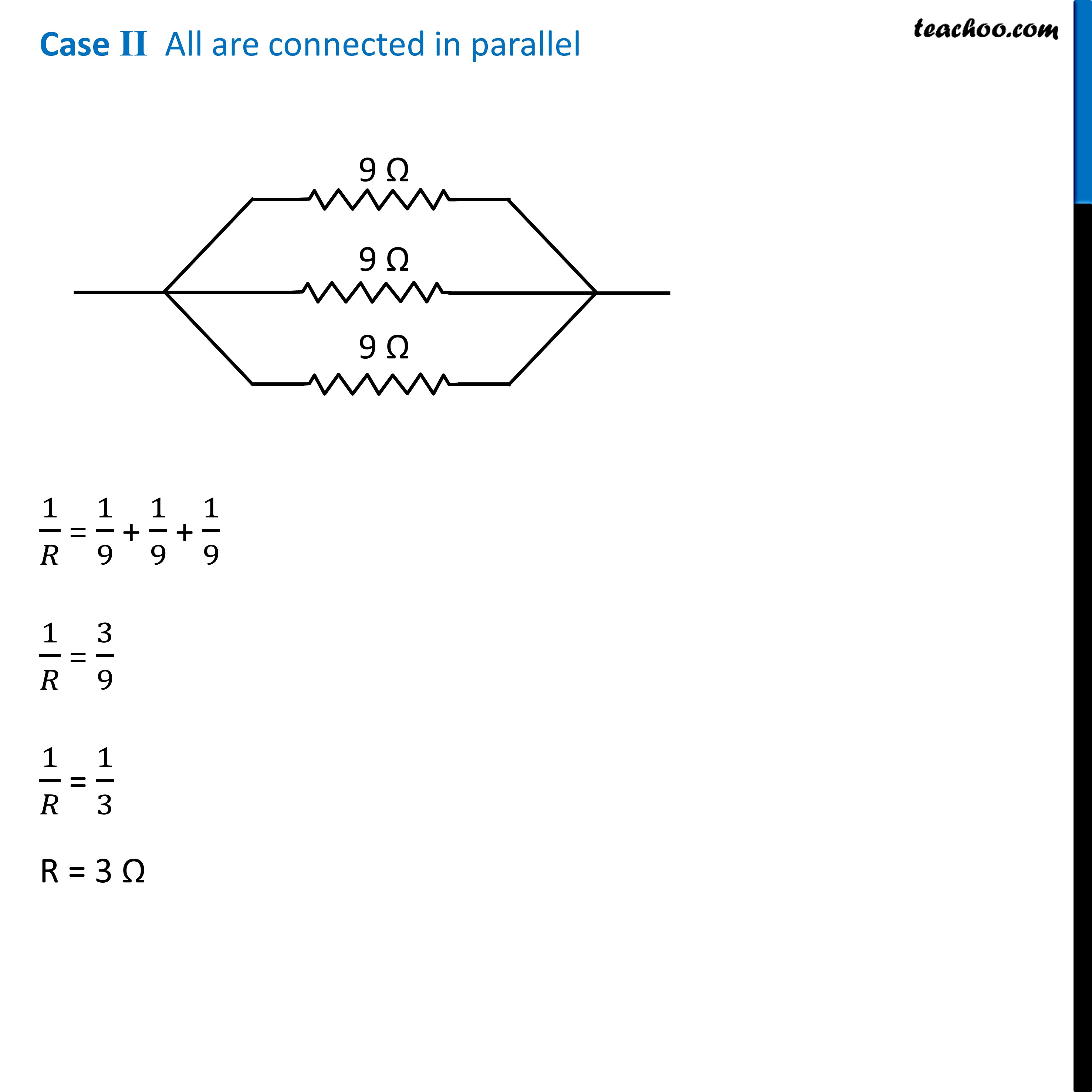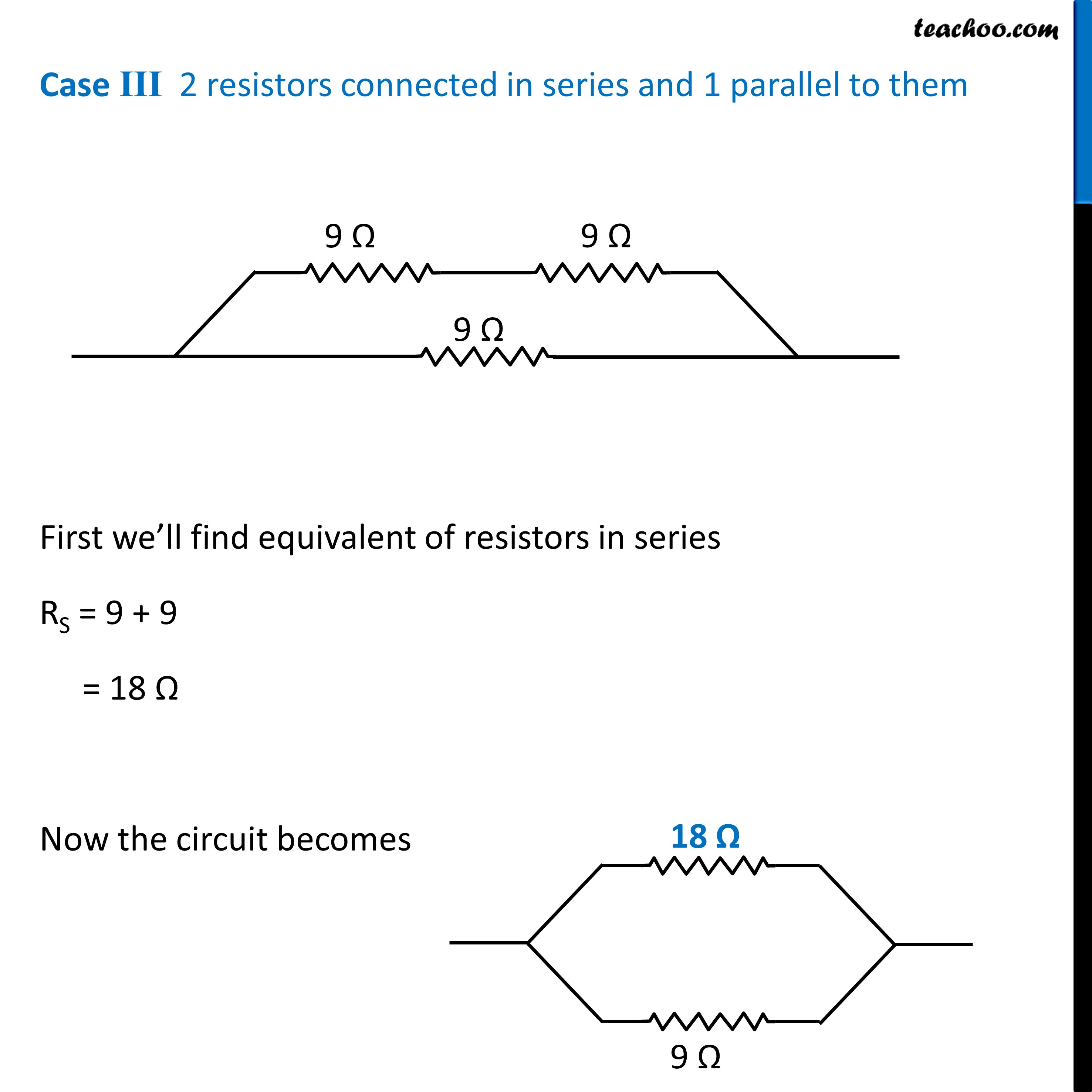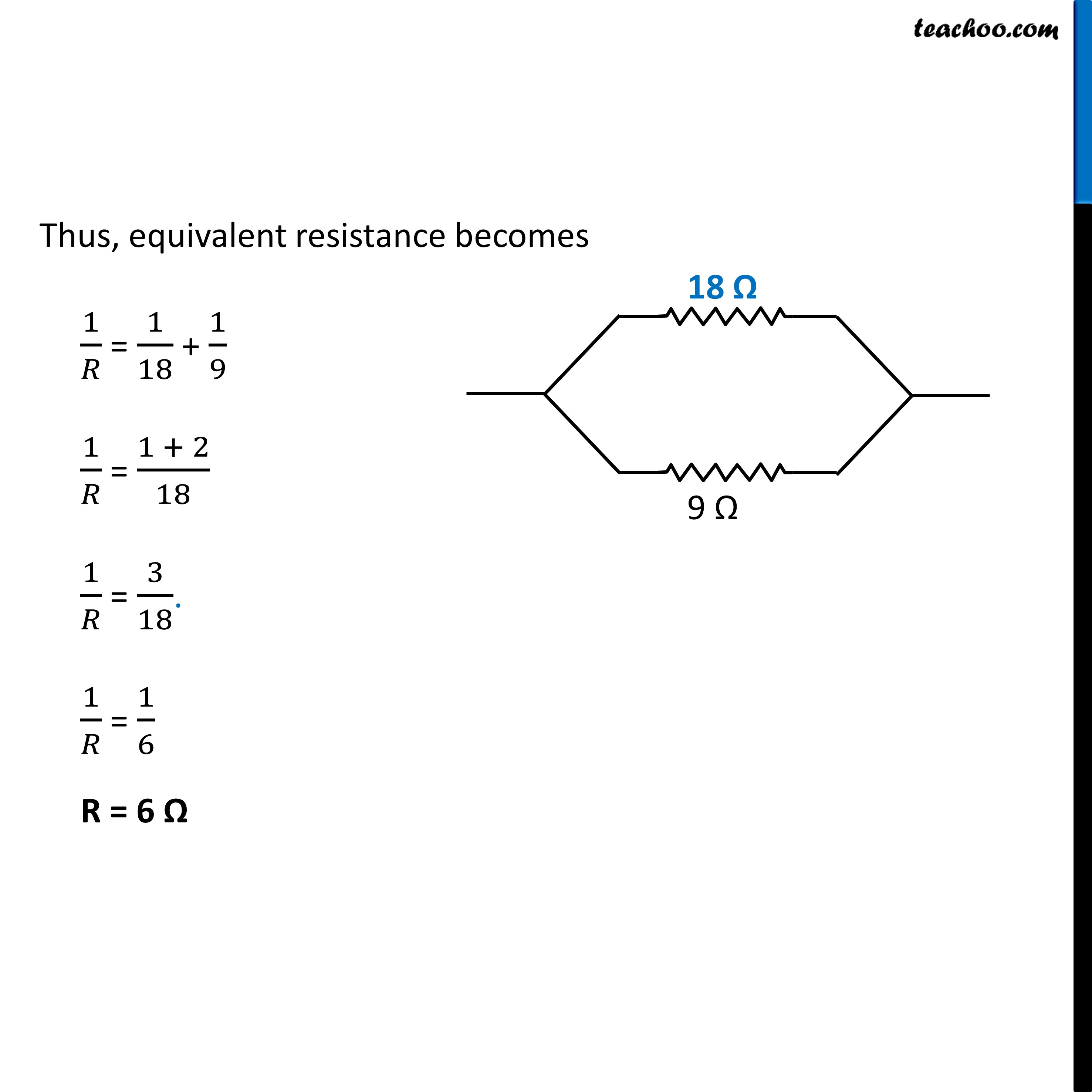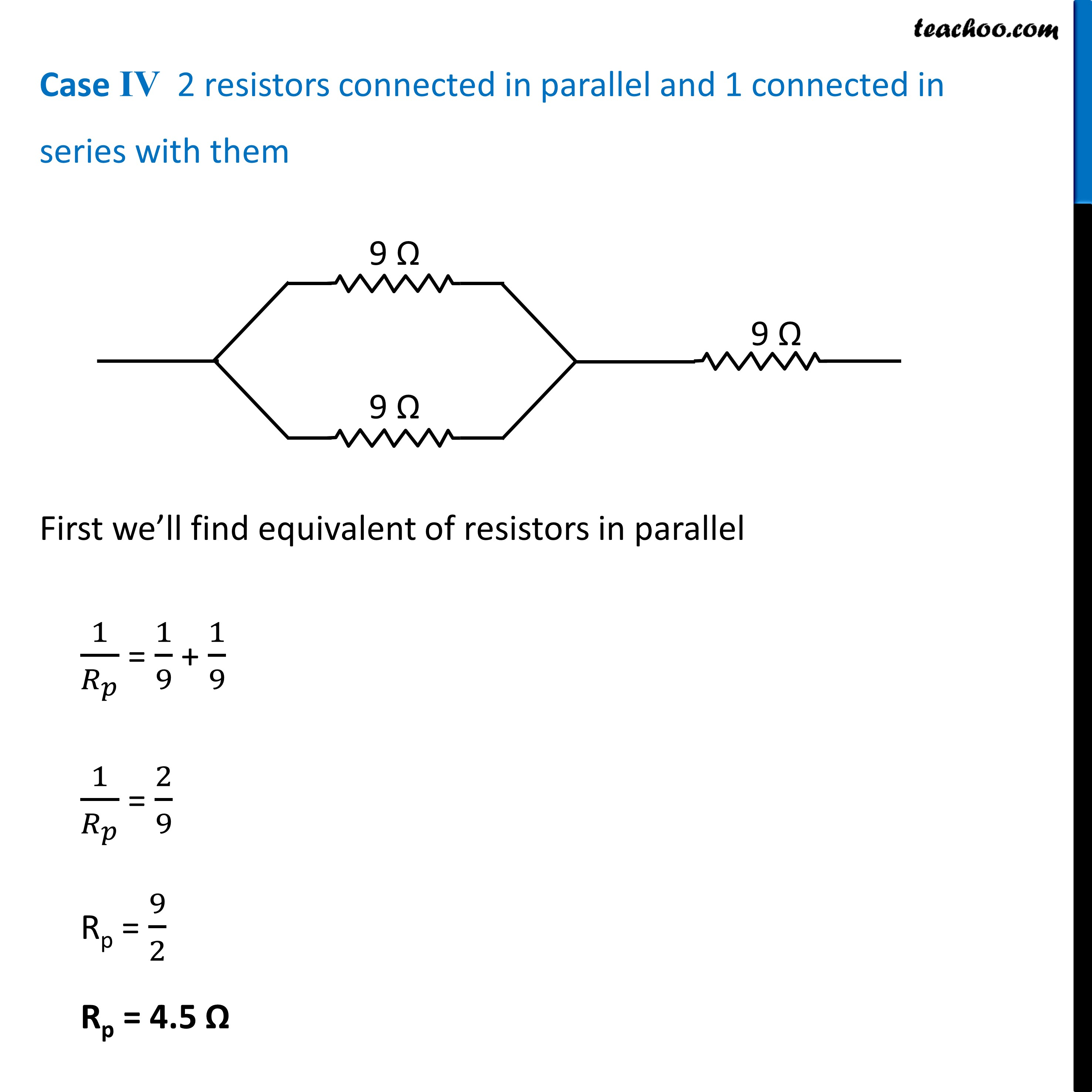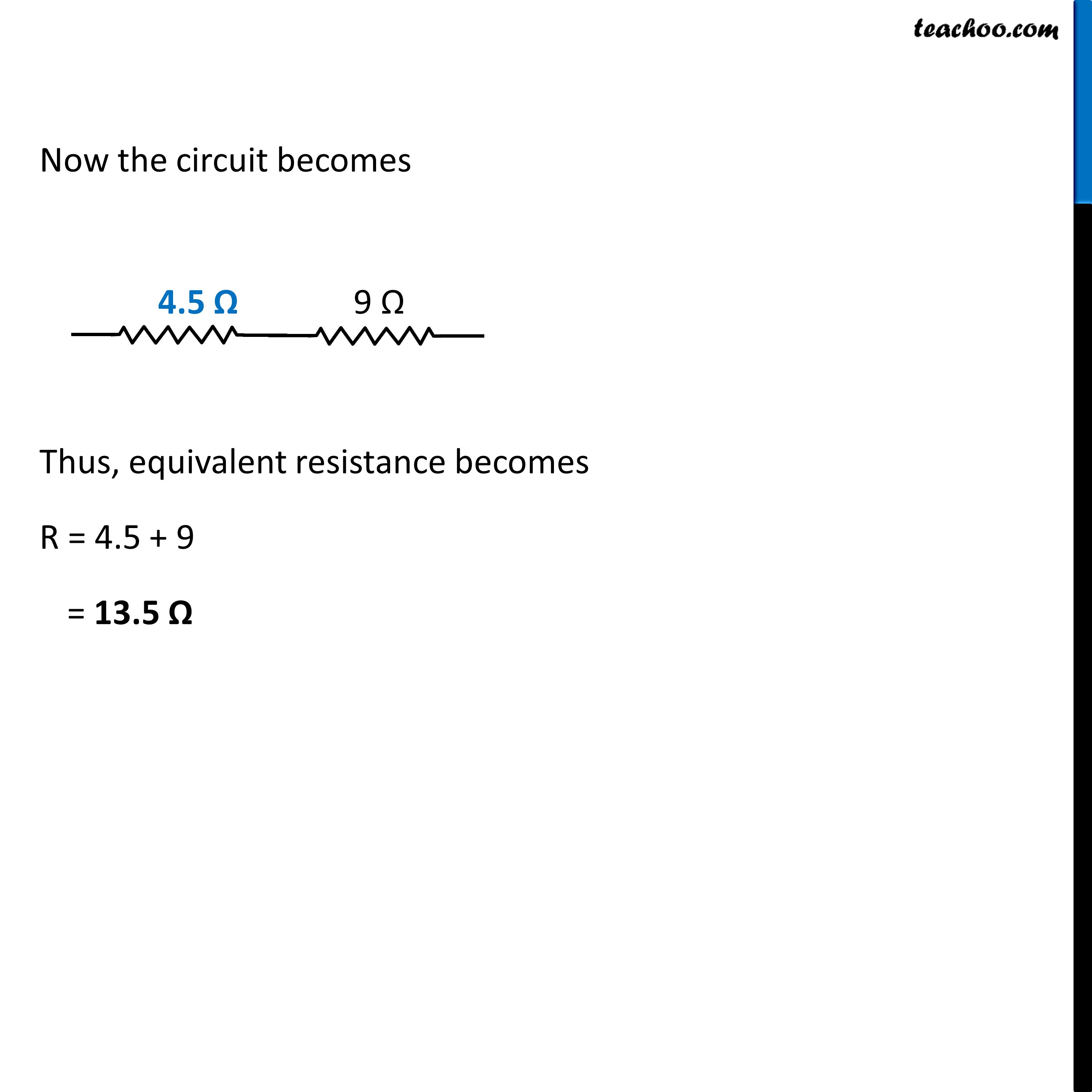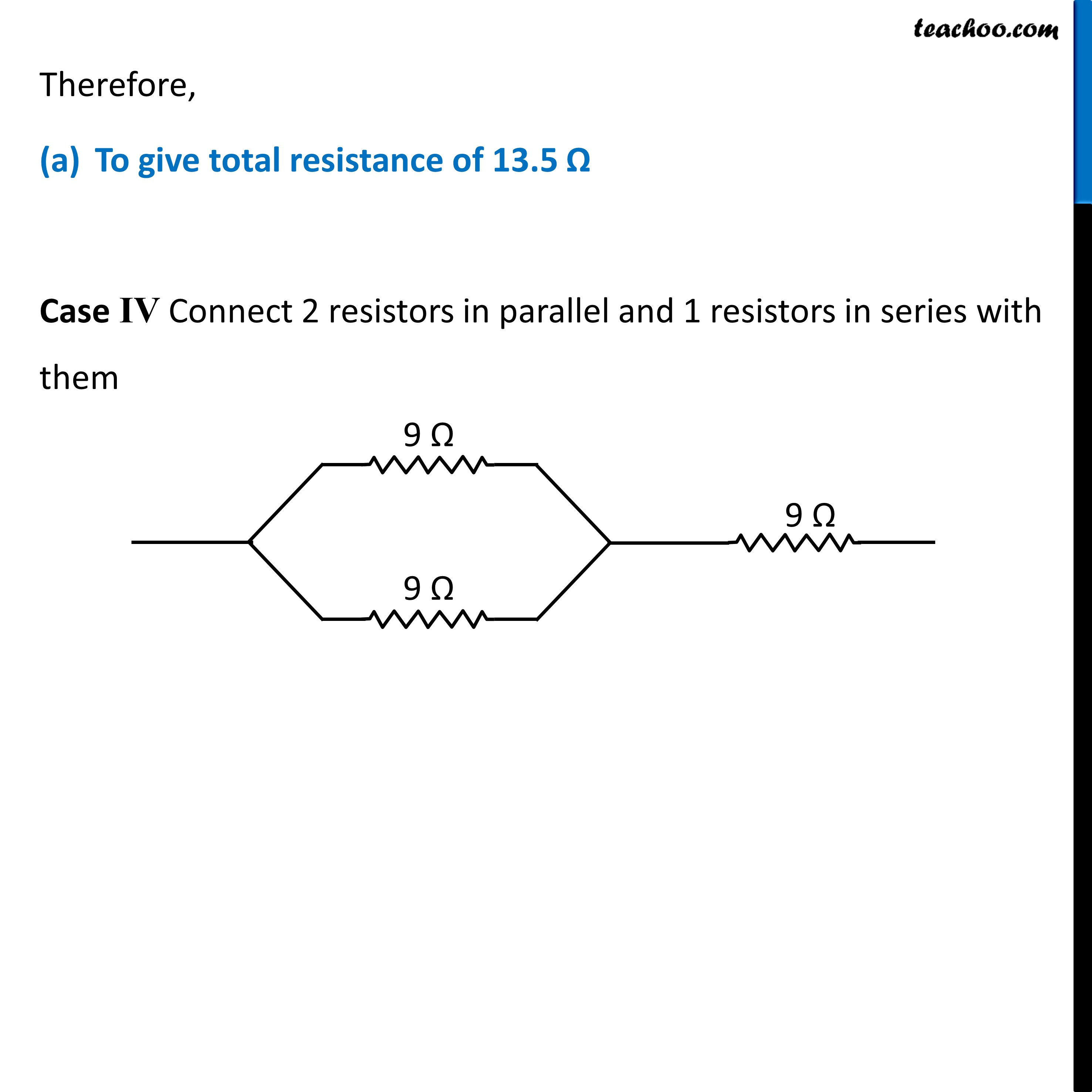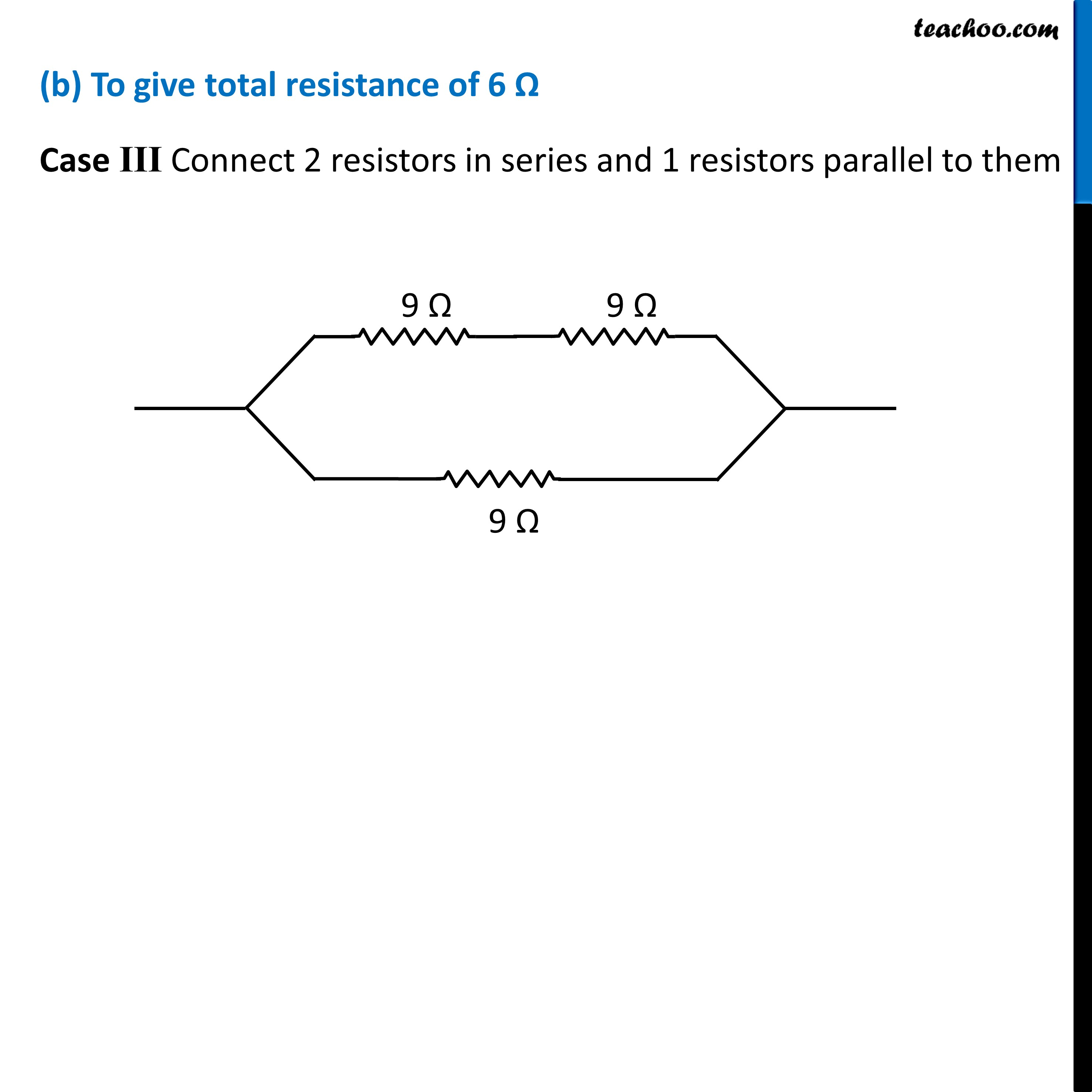Learn in your speed, with individual attention - Teachoo Maths 1-on-1 Class

### Transcript

Question 8 Show how would you join three resistors, each of resistance 9 Ω so that the equivalent resistance of the combination is (i) 13.5 Ω, (ii) 6 Ω? This can be done in 4 ways All 3 in series All 3 in parallel 2 in parallel, 1 in series 2 in series, 1 in parallel In series combination, equivalent resistance is given by Rs = R1 + R2 + R3 In parallel combination, equivalent resistance is given by 1/𝑅_𝑃 = 1/𝑅_1 + 1/𝑅_2 + 1/𝑅_3 Case I All are connected in series R = 9 + 9 + 9 = 27 Ω Case II All are connected in parallel 1/𝑅 = 1/9 + 1/9 + 1/9 1/𝑅 = 3/9 1/𝑅 = 1/3 R = 3 Ω Case III 2 resistors connected in series and 1 parallel to them First we’ll find equivalent of resistors in series RS = 9 + 9 = 18 Ω Now the circuit becomes Thus, equivalent resistance becomes 1/𝑅 = 1/18 + 1/9 1/𝑅 = (1 + 2)/18 1/𝑅 = 3/18. 1/𝑅 = 1/6 R = 6 Ω Case IV 2 resistors connected in parallel and 1 connected in series with them First we’ll find equivalent of resistors in parallel 1/𝑅_𝑝 = 1/9 + 1/9 1/𝑅_𝑝 = 2/9 Rp = 9/2 Rp = 4.5 Ω Now the circuit becomes Thus, equivalent resistance becomes R = 4.5 + 9 = 13.5 Ω Therefore, To give total resistance of 13.5 Ω Case IV Connect 2 resistors in parallel and 1 resistors in series with them (b) To give total resistance of 6 Ω Case III Connect 2 resistors in series and 1 resistors parallel to them